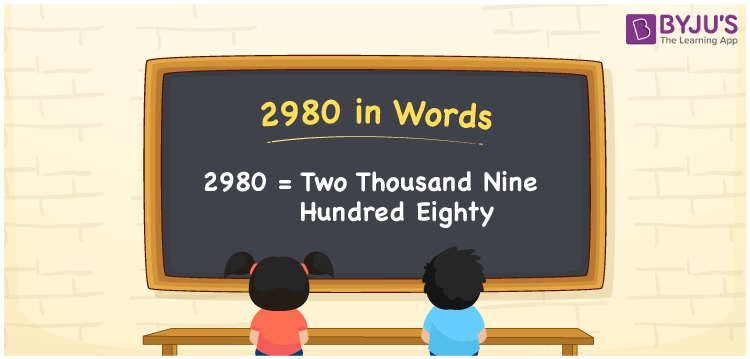# 2980 in Words

2980 in words is Two thousand nine hundred eighty. If you bought a new purse that costs Rs. 2980, then you can say, “I bought a new purse worth Two thousand nine hundred eighty rupees”. In this article, you will learn how to convert the numeral 2980 into words with the help of a place value chart, along with some interesting facts about 2980.

 2980 in words Two thousand nine hundred eighty Two thousand nine hundred eighty in Numbers 2980

## 2980 in English Words

We generally express numbers in words using the English alphabet. Thus, we can spell 2980 in English as “Two thousand nine hundred eighty”.## How to Write 2980 in Words?

Let us make a four-column place value chart to convert the number 2980 into words since it has four digits.

 Thousands Hundreds Tens Ones 2 9 8 0

Here, ones = 0, tens = 8, hundreds = 9, thousands = 2

These digits can be expanded as:

2 × Thousand + 9 × Hundred + 8 × Ten + 0 × One

= 2 × 1000 + 9 × 100 + 8 × 10 + 0 × 1

= 2000 + 900 + 80

= Two thousand + Nine hundred + Eighty

= Two thousand nine hundred eighty

Hence, 2980 in words = Two thousand nine hundred eighty.

As we know, 2980 is a natural number that precedes 2981 and succeeds 2979.

2980 in words – Two thousand nine hundred eighty

Is 2980 an odd number? – No

Is 2980 an even number? – Yes

Is 2980 a prime number? – No

Is 2980 a composite number? – Yes

Is 2980 a perfect square number? – No

Is 2980 a perfect cube number? – No

## Frequently Asked Questions on 2980 in Words

Q1

### How do you spell 2980?

We can spell the number 2980 in English words as Two thousand nine hundred eighty.
Q2

### How do you write Rs. 2980 in words on a cheque?

On a cheque, an amount of Rs. 2980 can be written in words as “Two thousand nine hundred eighty rupees only”.
Q3

### Write the value of 2980 + 4100 in words.

2980 + 4100 = 7080 We can write the value of 2980 + 4100, i.e., 7080 in words as Seven thousand and eighty.